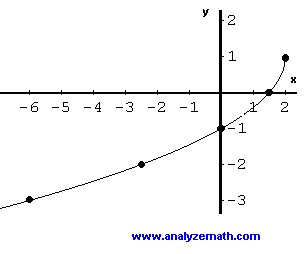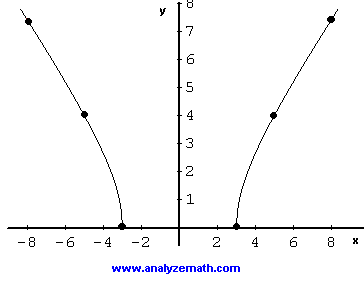# Graphing Square Root Functions

A step by step tutorial on graphing and sketching square root functions . The graph, domain, range and sometimes the simplifications of these functions and other properties are discussed.

## Review

The domain of function f defined by f(x) = √x is the set of all real positive numbers and zero because the square root of negative numbers are not real numbers (think of √ (- 4), is it real?). In inequality form, the domain of f(x) = √x is written as
x ≥ 0
in interval form the domain is given by
[ 0 , + ∞)

### Example 1

Make a table of values of function f given below, graph it and find its range.
f( x ) = √x

Solution to Example 1
Because the domain of f is the set of all positive real numbers and zero, we might construct a table of values as follows:
 x 0 1 4 9 16 √x 0 1 2 3 4

The values of x were selected so that the square root of these values are whole numbers which make it easy to plot the points shown in the table.The range of f is given by the interval [0 , +∞).

### Example 2

Find the domain, make a table of values of function f given below, graph it and find its range.
f( x ) = √ (x - 3)

Solution to Example 2
First find the domain of the square root function given above by stating that the expression under the square root must be positive or equal to zero
x - 3 ≥ 0
Solve the above inequality to obtain the domain of f as the set of all real values such that
x ≥ 3
We now select values of x in the domain to construct a table of values.
 x 3 4 7 12 √ (x - 3) 0 1 2 3The interval [0 , +∞) represents the range of f.

### Example 3

Find the domain, make a table of values of function f given below, graph it and find its range.
f( x ) = - √ (- 2x + 4) + 1

Solution to Example 3
The domain of the function given above is found by setting
- 2x + 4 ≥ 0
Solve the above inequality to obtain the domain of f as the set of all real values such that
x ≤ 2
We now select values of x in the domain of f to construct a table of values. These values are selected so that the square root term is a whole number and give points that are easy to plot.
 x 2 3/2 0 -5/2 -6 - √ (-2 x + 4 ) + 1 1 0 -1 -2 -3The range of f is given by the interval (-∞ , 1].

### Example 4

Find the domain of function f given below, graph it and find its range.
f( x ) = √ (- x 2 + 4)

Solution to Example 4
The domain of function given above is found by solving the polynomial inequality
- x
2 + 4 ≥ 0
The solution set of the above inequality is given by the interval
[-2 , 2]
which is also the domain of the above function.
Let us write the given function as an equation as follows
y = √ (- x
2 + 4)
Square both sides and arrange to obtain.
x
2 + y 2 = 2 2
The equation obtained is that of a circle. Hence the graph of f(x) = √ (- x 2 + 4) is the upper half of a circle sinsce √ (- x 2 + 4) is positive. Hence the graph below.The interval [0 , 2] represents the range of f.

### Example 5

Find the domain, make a table of values of function f given below, graph it and find its range.
f( x ) = √ (x 2 - 9)

Solution to Example 5
The domain of the function given above is found by solving
x
2 - 9 ≥ 0
Which gives a domain reprsented by
(-∞ , -3] U [3 , + ∞)
We now select values of x in the domain of f to construct a table of values, noting f(x) = f(-x) hence a symmetry of the graph with respect to the y axis.
 x 3 5 8 √ (x 2 - 9) 0 4 7.4The range of f is given by the interval [0 , + ∞).

### Example 6

Simplify f(x) given below, graph f and find its range.
f( x ) = √ (x 2 - 6x + 9)

Solution to Example 6
Let us use write the expression under the square root as a square as follows
x
2 - 6x + 9 = (x - 3) 2
Hence
f( x ) = √ (x
2 - 6x + 9)
= √ ( (x - 3)
2 ) = | x - 3 |
The given function has been rewitten as an absolute value function. Function f may be written as a piecewise function and graphed as follows.The range of f is given by the interval [0 , + ∞).

### Example 7

Graph the radicand (expression under the radical sign), make a table of values of function f given below, graph f and find its range..
f( x ) = √ (x 2 + 4x + 6)

Solution to Example 7
Use completing the square to rewtite the expression under the square root as follows
x
2 + 4x + 6 = (x + 2) 2 + 2
The expression under the square root is always positive hence the domain of f is the set of all real numbers. Let us first look at the graph of (x + 2) 2 + 2. It is a parabola.We would expect the graph of f to have the same axis of symmetry, the vertical line, x = -2 as the above graph. The table of values may constructed as follows.
 x -2 0 2 4 √ ( (x + 2) 2 + 2 ) 1.4 2.4 4.2 6.2The range of f is given by the interval [√2 , + ∞).

## More References and Links to Graphing

Graphing Functions